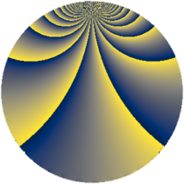# Properties

 Label 605.2.eLevel $605$ Weight $2$ Character orbit 605.e Rep. character $\chi_{605}(362,\cdot)$ Character field $\Q(\zeta_{4})$ Dimension $92$ Newform subspaces $3$ Sturm bound $132$ Trace bound $1$

# Related objects

## Defining parameters

 Level: $$N$$ $$=$$ $$605 = 5 \cdot 11^{2}$$ Weight: $$k$$ $$=$$ $$2$$ Character orbit: $$[\chi]$$ $$=$$ 605.e (of order $$4$$ and degree $$2$$) Character conductor: $$\operatorname{cond}(\chi)$$ $$=$$ $$55$$ Character field: $$\Q(i)$$ Newform subspaces: $$3$$ Sturm bound: $$132$$ Trace bound: $$1$$ Distinguishing $$T_p$$: $$2$$

## Dimensions

The following table gives the dimensions of various subspaces of $$M_{2}(605, [\chi])$$.

Total New Old
Modular forms 156 124 32
Cusp forms 108 92 16
Eisenstein series 48 32 16

## Trace form

 $$92q + 8q^{3} + 8q^{5} + O(q^{10})$$ $$92q + 8q^{3} + 8q^{5} - 36q^{12} + 12q^{15} - 20q^{16} - 16q^{20} - 36q^{23} - 20q^{25} + 48q^{26} - 4q^{27} - 8q^{31} - 36q^{36} - 4q^{37} - 40q^{38} + 60q^{42} + 8q^{45} + 20q^{47} - 64q^{48} - 4q^{53} - 32q^{56} + 64q^{58} + 152q^{60} - 68q^{67} + 16q^{70} + 48q^{71} - 28q^{75} - 84q^{78} - 112q^{80} + 68q^{81} - 44q^{82} + 8q^{86} - 16q^{91} - 20q^{92} - 76q^{93} + 56q^{97} + O(q^{100})$$

## Decomposition of $$S_{2}^{\mathrm{new}}(605, [\chi])$$ into newform subspaces

Label Dim. $$A$$ Field CM Traces $q$-expansion
$$a_2$$ $$a_3$$ $$a_5$$ $$a_7$$
605.2.e.a $$20$$ $$4.831$$ $$\mathbb{Q}[x]/(x^{20} + \cdots)$$ None $$0$$ $$4$$ $$4$$ $$0$$ $$q+\beta _{1}q^{2}-\beta _{12}q^{3}+(-\beta _{4}+\beta _{10}-\beta _{13}+\cdots)q^{4}+\cdots$$
605.2.e.b $$32$$ $$4.831$$ None $$0$$ $$-4$$ $$8$$ $$0$$
605.2.e.c $$40$$ $$4.831$$ None $$0$$ $$8$$ $$-4$$ $$0$$

## Decomposition of $$S_{2}^{\mathrm{old}}(605, [\chi])$$ into lower level spaces

$$S_{2}^{\mathrm{old}}(605, [\chi]) \cong$$ $$S_{2}^{\mathrm{new}}(55, [\chi])$$$$^{\oplus 2}$$